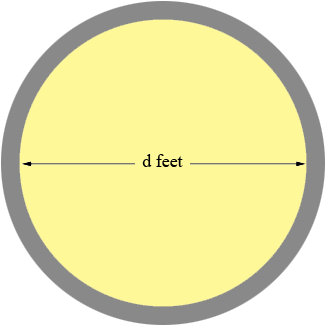SEARCH HOMEMath Central Quandaries & QueriesQuestion from John: I need to find the inside diameter of a cement silo with an outside circumference 118 ft and a wall thickness of 7 inches. thank you John.Hi John,

The circumference of a circle is given by $\pi$ times the diameter. Suppose the inside diameter is $d$ feet.Then, since the thickness of the wall is $7$ inches which is $\large \frac{7}{12}$ feet, the outside diameter is

$d + 2 \times \frac{7}{12} \mbox{ feet.}$

But the outside circumference is 118 feet and hence

$\pi \left( d + 2 \times \frac{7}{12} \right) = 118$

and thus

$d + 2 \times \frac{7}{12} = \frac{118}{\pi}.$

Finally

$d = \frac{118}{\pi} - 2 \times \frac{7}{12} = 36.4 \mbox{ feet.}$

PennyMath Central is supported by the University of Regina and The Pacific Institute for the Mathematical Sciences.